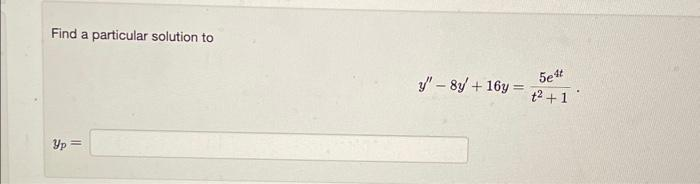# Question Find a particular solution to $y^{\prime \prime}-8 y^{\prime}+16 y=\frac{5 e^{4 t}}{t^{2}+1}$ $y_{p}=$Transcribed Image Text: Find a particular solution to $y^{\prime \prime}-8 y^{\prime}+16 y=\frac{5 e^{4 t}}{t^{2}+1}$ $y_{p}=$
Transcribed Image Text: Find a particular solution to $y^{\prime \prime}-8 y^{\prime}+16 y=\frac{5 e^{4 t}}{t^{2}+1}$ $y_{p}=$